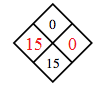### Home > CC2MN > Chapter 7 > Lesson 7.2.3 > Problem7-85

7-85.

Copy and complete each of the Diamond Problems below. The pattern used in the Diamond Problems is shown at right.1.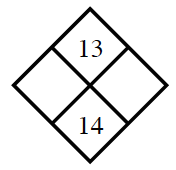What are the factors of $13$?

The sum of which two factors is $14$?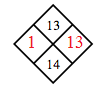1.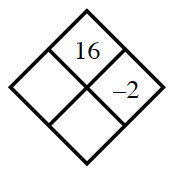What is $16$ divided by $-2$?

1.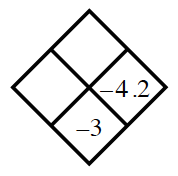What can you add to $-4.2$ to get a sum of $-3$?

1.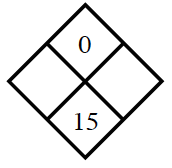Since the product is $0$, at least one of the missing numbers must be $0$.